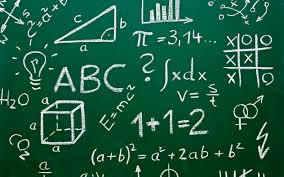# Math

###General Math | Algebra| Elementary Level Math | Geometry| Math Tables, Definitions and Other Cool Stuff

General Math

Dr. Math to the rescue

Math Stories.com
Very nice site with word problems for students grades 1-5

Math.com Homework Help
Great help with pre-algebra, algebra and geometry

AAA Math
If you want to have fun learning math, then try this site

Math for Morons Like Us
Don’t be insulted by the title…it is really cool!

Cool Math.com
Definitely cool!

Cool Math for Kids
Terrific kids math site…Sometimes math CAN be fun

Math Homework Helper
Great math help for elementary and middle school students from Fact Monster

Frank Potter’s Science Gems – Mathematics
Don’t miss Mr. Potter’s sites

The Math Forum
Wonderful math page with many math links and information

Topics in Mathematics Math Archives
You will find just about any kind of math here

Harcourt Animated Math Glossary
A definitely cool glossary of math terms

Mathwords

Math definitions from algebra through calculus

Print Free Graph Paper

This site is cool…you can print all kinds of graph paper

Algebra

Ask Dr. Math High School Algebra

Dr. Math has been around for years

Ask Dr. Math Middle School Algebra

What can I say…he’s great

Math is Fun – Algebra

This site is really good

Math10.com Algebra

Really nice presentation of Algebra

Introduction to Algebra
Nice intro site

Algebra Worksheet Generator
Want to make your own algebra worksheets?

Free Algebra 1 Worksheets

Lots of worksheets here…very nice

Elementary Level Math

Dr. Math rescues elementary school students…Hooray Dr. Math

This site is great fun for elementary and middle school students

Electronic Flash Cards for Kids
Learn math the with electronic flash cards…great for kids

Math Dictionary for Kids
Great math dictionary for kids

Superkids Math Worksheets
Creates math problems for elementary and middle school students…don’t miss this one

Geometry

Geometry Formulas and Facts
Nice geometry site

Geometry Center Search Page
Type in a geometry term and watch the results…WOW!

Math.com Geometry

Math.com is really good

Math10.com Geometry

Oh you will really love this site…clear presentation

Basics in Geometry
Wonderful introductory site to geometry…must see!

The Geometry Junkyard
Even math haters love this site

Math is Fun – Geometry

Even if you don’t like geometry, you’ll like this site

Math Tables, Definitions and Other Cool Stuff

Math2.org
Don’t miss this site with all kinds of math formulas

Hotmath
Check to see if they have your math textbook…a must see site!

Math Index
A terrific index of math terms from George Mason University

Favorite Mathematical Constants
Pi, Logarithms, etc. –Great site for serious math students

Save

Save

Save

Save

Save

Save

Save

Save

Save

Save